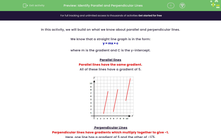# Identify Parallel and Perpendicular Lines

In this worksheet, students will understand the relationship between the gradients of parallel and perpendicular lines.Key stage:  KS 3

Curriculum topic:   Algebra

Curriculum subtopic:   Use Linear Equations (Two Variables) and Gradients

Difficulty level:#### Worksheet Overview

In this activity, we will build on what we know about parallel and perpendicular lines.

We know that a straight line graph is in the form:

## y = mx + c

where m is the gradient and C is the y-intercept.

Parallel lines

Parallel lines have the same gradient.

All of these lines have a gradient of 5.Perpendicular Lines

Perpendicular lines have gradients which multiply together to give -1.

Here, one line has a gradient of 5 and the other of -1/5.To find the gradient of a line which is perpendicular to one of gradient a/b, we invert the fraction and multiply by -1.

This is called the 'negative reciprocal'.Don't worry - it's easier than it sounds!

We are going to use all this information to find straight lines which are parallel and perpendicular!

Let's look at a typical question!

Example

State the straight line which is parallel to

### y = 2x + 5

and goes through the point (0, 3)

From the straight line

### y = 2x + 5

We can see that the gradient m is 2

For a line to be parallel, it must have the same gradient.

y = 2x + C will be parallel.

Our line also passes through (0, 3) which is the y-intercept C

y = 2x + 3

We do exactly the same for a perpendicular line, but instead of the gradient m repeated we find the negative reciprocal.

That is the inverse and x by -1

So, if the gradient was 2, then the perpendicular gradient is -1/2Let's try some questions!

### What is EdPlace?

We're your National Curriculum aligned online education content provider helping each child succeed in English, maths and science from year 1 to GCSE. With an EdPlace account you’ll be able to track and measure progress, helping each child achieve their best. We build confidence and attainment by personalising each child’s learning at a level that suits them.

Get started